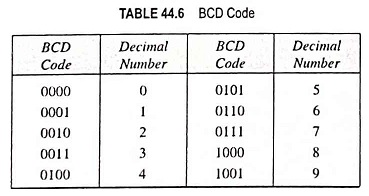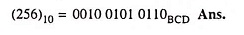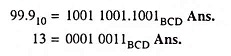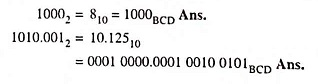## What is Binary Coded Decimal (BCD) Code?:

The 8-4-2-1 code or simply the Binary Coded Decimal code is composed of four bits representing the decimal digits 0 through 9, 8, 4, 2 and 1 are the weights of the four bits (23, 22, 21, 20) of each decimal digit similar to straight binary number system. The ease of conversion between 8-4-2-1 code numbers and familiar decimal numbers is the main advantage of this code.

The BCD code means that each decimal digit is represented by a binary code of four bits. Clearly only the 4-bit binary numbers from 0000 through 1001 are used. The Binary Coded Decimal code does not use the numbers 1010, 1011, 1100, 1101, 1110, and 1111. In other words, only 10 of the sixteen (24) possible 4-bit binary code groups are used. If any of the ‘forbidden’ 4-bit number ever occurs in a machine using the Binary Coded Decimal code, it is usually an indication that an error has occurred.

Any decimal number can be expressed in BCD code by replacing each decimal digit by the appropriate 4-bit combinations. Ten binary combinations that represent the ten decimal digits are shown in Table 44.6. Conversely, a BCD number can be easily converted into a decimal number by dividing the coded number into groups of 4-bits (starting with LSB) and then writing down the decimal digit represented by each four-bit group.It is very important to realize that BCD is not another number system like binary, octal, decimal, and hexadecimal. It is, in fact, the decimal system with each decimal digit encoded in its binary equivalent. It is also important to note that a BCD number is not the same number as a straight binary number. A straight binary code takes the complete decimal number and represents it in binary while the Binary Coded Decimal code converts each decimal digit to binary individually.

Binary Coded Decimal code is a numerical code, and many applications need that arithmetic operations be carried out. Addition is the most important operation because the other three operations (subtraction, multiplication and division) can be accomplished using addition. For adding two BCD numbers :

2. If a four-bit sum is equal to 9 or less than 9, it is a valid BCD number.
3. If a four-bit sum exceeds 9, or if a carry-out of the group is generated, it is an invalid result. Add 6 (0110)2 to the four-bit sum. If a carry results when (0110)2 is added, simply add the carry to the next four-bit group.

### BCD Subtraction:

For subtracting the two numbers:

1. Find the 9’s complement of the decimal subtrahend and encode that number in BCD code.
2. The resulting BCD number is added as described above.

Example 44.65: Convert the following number decimal number (256)10 to its BCD equivalent.

Solution:Example 44.66: Convert the following decimal numbers into 8-4-2-1 BCD equivalents.

Solution:Example 44.67: Convert the following binary into their 8-4­-2-1 BCD equivalent.

Solution:Scroll to Top